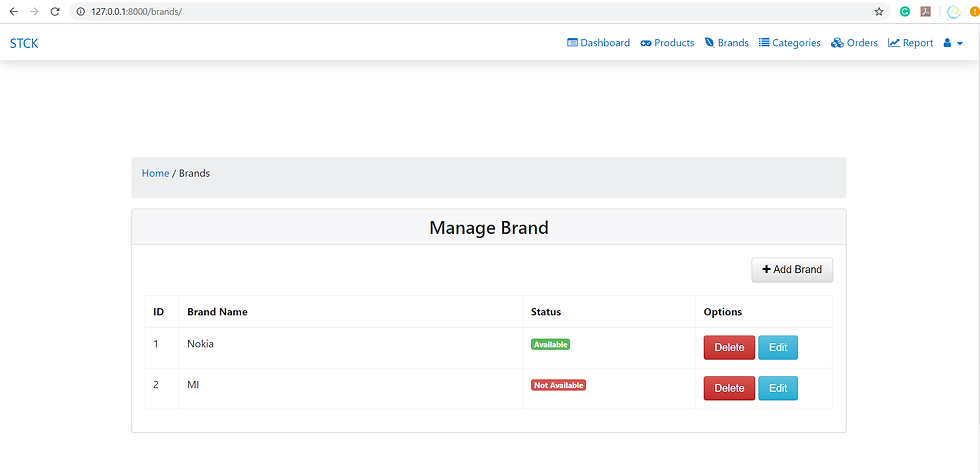top of page
Search

# Django: Stock Management System | Python Web Assignment Help | CodersartsDjango is a high-level Python web development framework. It is used to create a secure web application in python. If you are looking for any project assignment related help then you can contact us and get any assignment related help immediately.

Our expert is well educated and providing help when you need it.

In this blog, we will go through the front view of the "Stock Management App".

### Front Views of Stock Management AppStep1:

Open editor: pycharm

Step2:

Create project “stock” using

`>django-admin startproject stock`

Step3:

Create app “app” after change directory to “stock”

```./ems> python manage.py startapp app
```

Step4:

`>python manage.py createsuperuser`

Step5:

Apply two-step migrations

```>python manage.py makemigrations
>python manage.py migrate```

Step6:

Run using

`>python manage.py runserver`As per the above steps, you can create another app that I need to create this app.

Here some screenshots of the output result.

Here code overview:

urls.py:

```
from django.urls import path, include```

```
urlpatterns = [
path('', include("app.urls")),
]```

models.py

```from django.db import models

CHOICES = (
("1", "Available"),
("2", "Not Available")
)

class Brand(models.Model):
name = models.CharField(max_length=255)
status = models.CharField(max_length=10, choices=CHOICES)

def __str__(self):
return self.name

class Category(models.Model):
name = models.CharField(max_length=255)
status = models.CharField(max_length=10, choices=CHOICES)

def __str__(self):
return self.name

class Product(models.Model):

name = models.CharField(max_length=255)
code = models.CharField(max_length=10)
quantity = models.IntegerField()
rate = models.FloatField(max_length=100)
status = models.CharField(max_length=10, choices=CHOICES)

def __str__(self):
return self.name

class Order(models.Model):
sub_total = models.FloatField(max_length=100)
vat = models.FloatField(max_length=100)
total_amount = models.FloatField(max_length=100)
discount = models.FloatField(max_length=100)
grand_total = models.FloatField(max_length=100)
paid = models.FloatField(max_length=100)
due = models.FloatField(max_length=100)
payment_type = models.CharField(max_length=100)
payment_status = models.IntegerField()
status = models.IntegerField()

class OrderItem(models.Model):

quantity = models.IntegerField()
rate = models.FloatField(max_length=100)
total = models.FloatField(max_length=100)
status = models.IntegerField()```If you need any project Assignment related to Django, then you can contact us at contact@codersarts.com